# CBSE Class 12 Chemistry Solved Sample Paper 2021-22 (Term-1)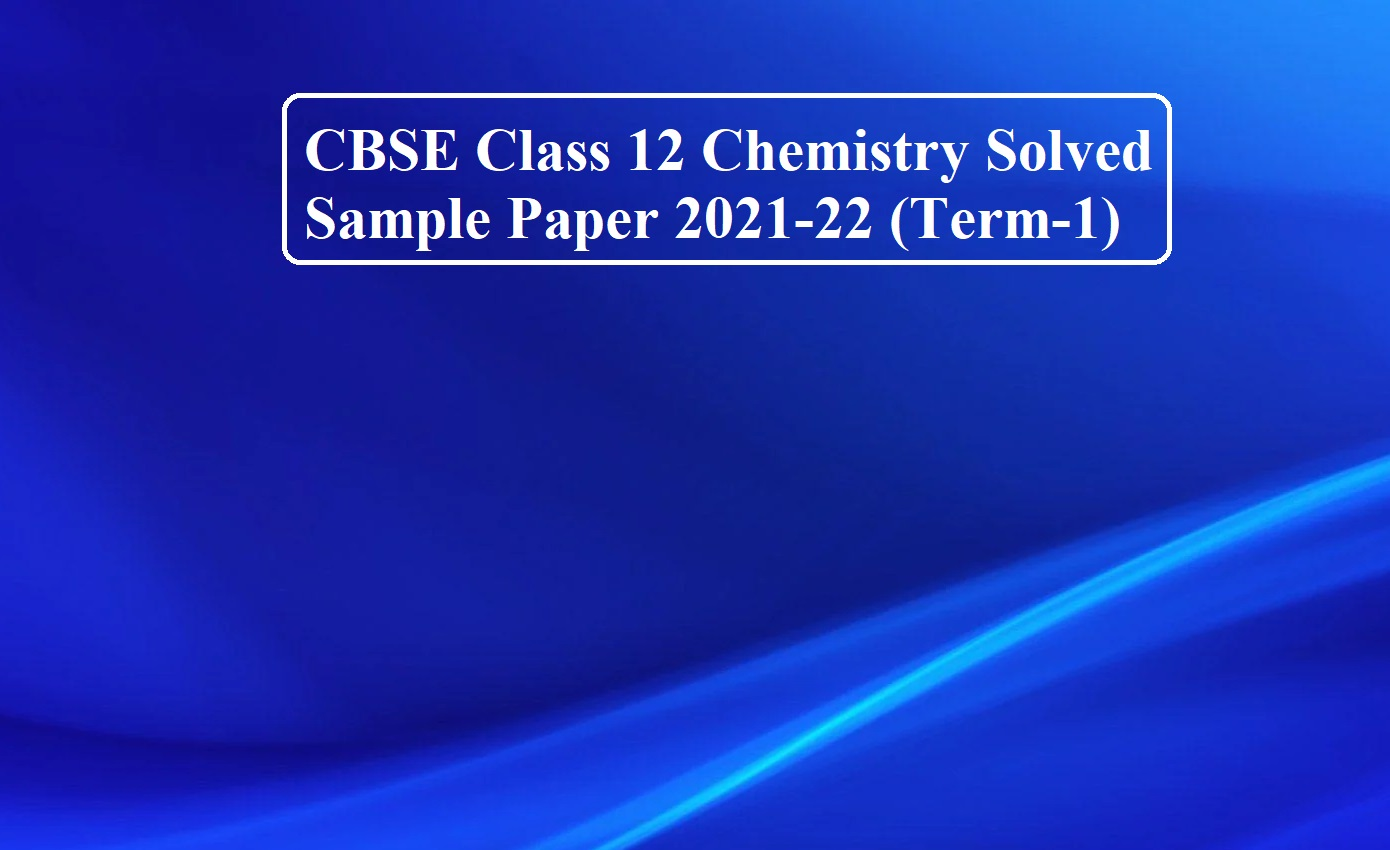CBSE Class 12 Chemistry Solved Sample Paper 2021-22 (Term-1)

CBSE Class 12 Chemistry Solved Sample Paper 2021-22 (Term-1)

Subject: Chemistry (043)

Time: 90 Minutes                                                          Max. Marks: 35

Steven Wright, Known Wife Beater, Gets Suspended 80 Games For Doing Steroids – Deadseriousness anadrol 50 prices activity – which anabolic steroid is best for bodybuilding, which anabolic steroids to take – playarcade.fun
General Instructions:

1. The Question Paper contains three sections.
2. Section A has 25 questions. Attempt any 20 questions.
3. Section B has 24 questions. Attempt any 20 questions.
4. Section C has 6 questions. Attempt any 5 questions.
5. All questions carry equal marks. 6. There is no negative marking.

SECTION A

This section consists of 25 multiple choice questions with an overall choice to attempt any 20 questions. In case more than a desirable number of questions are attempted, ONLY the first 20 will be considered for evaluation.

1. Which of the following statements is true:
(a) Melting point of Phosphorous is less than that of Nitrogen
(b) N2 is highly reactive while P4 is inert
(c) Nitrogen shows a higher tendency of catenation than P
(d) N-N is weaker than P-P
Ans 1. (d) N-N is weaker than P-P
Reason: Other statements as incorrect as Phosphorus has a higher melting point due to its bigger size than Nitrogen. Nitrogen is inert due to the formation of triple bonds and has a lower covalence due to non – availability of d –orbitals

2. Which of the following is a non-stoichiometric defect?
(a) Frenkel defect
(b) Schottky defect
(c) Metal deficiency defect
(d) Interstitial defect
Ans 2. (c) Metal deficiency defect (anion is missing from lattice site)
Reason: In the Frenkel defect, the smaller ion occupies the interstitial sites and the Schottky defect equal number of cations and anions are missing. Interstitial defect an atom or molecule occupies intestinal sites so in these three defects the ratio of positive and negative ions (Stoichiometric) of a solid is not disturbed in these three

3. Identify the law which is stated as:
“For any solution, the partial vapour pressure of each volatile component in the solution is directly proportional to its mole fraction.”
(a) Henry’s law
(b) Raoult’s law
(c) Dalton’s law
(d) Gay-Lussac’s Law
Ans 3. (b) Raoult’s law

4. Pink colour of LiCl crystals is due to:
(a) Schottky defect
(b) Frenkel defect
(c) Metal excess defect
(d) Metal deficiency defect
Ans 4. (c) Metal excess defect (formation of F centres)

5. Which of the following isomer has the highest melting point:
(a) 1,2-dichlorobenzene
(b) 1,3 -dichlorobenzene
(c) 1,4-dichlorobenzene
(d) All isomers have the same melting points
Ans 5. (c) 1,4-dichlorobenzene (para isomers are more symmetric and ortho and meta)

CBSE Class 12 Chemistry Solved Sample Paper 2021-22 (Term-1)

6. Which one of the following reactions is not explained by the open-chain Structure of glucose:
(a) Formation of pentaacetate of glucose with acetic anhydride.
(b) Formation of addition product with 2,4 DNP reagent
(c) Silver mirror formation with Tollen’s reagent
(d) Existence of alpha and beta forms of glucose.
Ans 6. (d) Existence of alpha and beta forms of glucose.

7. Williamson’s synthesis of preparing dimethyl ether is an:
(a) SN1 reaction
(b) Elimination reaction
(c) SN2 reaction
Ans 7. (c) SN2 reaction (alkoxide ion reacts with a primary alkyl halide in a single step to form ether)

8. Chlorine water loses its yellow colour on standing because:
(a) HCl gas is produced, due to the action of sunlight.
(b) A mixture of HOCl and HCl is produced in the presence of light
(c) HOCl and hydrogen gas is produced
(d) A mixture of HCl and ClO3 is produced, due to the action of sunlight
Ans 8. (b) A mixture of HOCl and HCl is produced in the presence of sunlight
Cl2(g) + H2O (l) → HCl (g) + HOCl(aq)

9. During dehydration of alcohols to alkenes by heating with concentrated H2SO4, the initiation step is:
(a) Protonation of an alcohol molecule
(b) Formation of carbocation
(c) Elimination of water
(d) Formation of an ester
Ans 9. (a) Protonation of alcohol molecule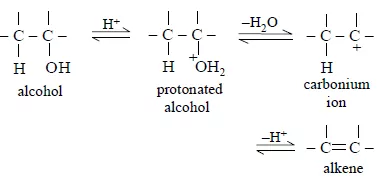10. Amorphous solids are:
(a) Isotropic
(b) Anisotropic
(c) Isotopic
(d) Isomeric
Ans 10. (a) Isotropic (the value of any physical property is the same along any direction)

11. Which of the following reactions is used to prepare salicylaldehyde?
(a) Kolbe’s reaction
(b) Etard reaction
(c) Reimer – Tiemann reaction
(d) Stephen’s reduction.
Ans 11. (c) Reimer- Tiemann reaction ( Kolbe’s reaction is used to prepare salicylic acid, Etard reaction for benzaldehyde, Reimer- Tiemann reaction for salicylaldehyde and Stephen’s reduction for aldehyde)

12. Which of the following is an example of a solid solution?
(a) Sea-water
(b) Sugar solution
(c) Smoke
(d) 22-carat gold
Ans 12. (d) 22-carat gold (it is an alloy so solid in solid solution

13. The boiling points of alcohols are higher than those of hydrocarbons of comparable masses due to:
(a) Hydrogen bonding
(b) Ion – dipole interaction
(c) Dipole- dipole interaction
(d) Van der Waal’s forces.
Ans 13. (a) Hydrogen bonding (alcohols form intermolecular hydrogen bonds)

14. Which of the following has the lowest boiling point:
(a) H2O
(b) H2S
(c) H2Se
(d) H2Te
Ans 14. (b) H2S (boiling point increases down the group but water forms strong hydrogen bonds so has a higher boiling point than H2S)

15. Which of the following statement is correct:
(a) Fibrous proteins are generally soluble in water
(b) Albumin is an example of fibrous proteins
(c) In fibrous proteins, the structure is stabilized by hydrogen bonds and disulphide bonds
(d) pH does not affect the primary structure of a protein.
Ans 15. (d) pH does not affect the primary structure of the protein (pH affects the tertiary structure)

16. Major product obtained on the reaction of 3-Phenyl propene with HBr in presence of organic peroxide
(a) 3- Phenyl 1- bromopropane
(b) 1 –Phenyl -3- bromopropane
(c) 1-Phenyl -2-bromopropane
(d) 3-Phenyl -2- bromopropane
Ans 16. (b) 1 – Phenyl – 3 – bromopropane
((C6H5)CH2CH=CH2 + HBr (organic peroxide) → (C6H5)CH2CH2CH2Br Anti-Markovnikov addition)

17. Which of the following is a correct statement for C2H5Br?
(a) It reacts with metallic Na to give ethane.
(b) It gives nitroethane on heating with an aqueous solution of AgNO2
(c) It gives C2H5OH on boiling with alcoholic potash.
(d) It forms diethylthioether on heating with alcoholic KSH.
Ans 17. (b) It gives nitroethane on heating with aqueous solution of AgNO2
(C2H5Br reacts with metallic Na to give butane , gives ethene on boiling with alcoholic potash and forms C2H5SH (thiol) on heating with alcoholic KSH)

18. Covalency of nitrogen is restricted to:
(a) 2
(b) 3
(c) 4
(d) 5
Ans 18. (c) 4 (Covalency of nitrogen is restricted to 4 due to non availability of d – orbitals)

19. Solubility of gases in liquids decreases with rising in temperature because dissolution is an:
(a) Endothermic and reversible process
(b) Exothermic and reversible process
(c) Endothermic and irreversible process
(d) Exothermic and irreversible process
Ans 19. (b) Exothermic and reversible process (according to Le -Chatlier principle Solubility of gases in liquids decreases with rise in temperature)

20. All elements of Group 15 show allotropy except:
(a) Nitrogen
(b) Arsenic
(c) Antimony
(d) Bismuth
Ans 20. (a) Nitrogen (due to small size and high electronegativity N-N is weak).

CBSE Class 12 Chemistry Solved Sample Paper 2021-22 (Term-1)

21. Which of the following is a polysaccharide?
(a) Glucose
(b) Maltose
(c) Glycogen
(d) Lactose
Ans 21. (c) Glycogen (It is a polymer of glucose)

22. Substance having the lowest boiling point:
(a) Hydrogen
(b) Oxygen
(c) Nitrogen
(d) Helium
Ans 22. (d) Helium (He is monoatomic and has low atomic mass)

23. Lower molecular mass alcohols are:
(a) Miscible in a limited amount of water
(b) Miscible in excess of water
(c) Miscible in water in all proportions
(d) Immiscible in water
Ans 23. (c) miscible in water in all proportions Lower molecular mass alcohols are able to form hydrogen bonds with water

24. Maximum oxidation state exhibited by Chlorine is:
(a) +1
(b) +3
(c) +5
(d) +7
Ans 24. (d) +7 (Cl : 1s2 2s2 2p6 3s2 3p5). Due to 7 valence electrons.

25. In which of the following cases blood cells will shrink:
(a) When placed in water containing more than 0.9% (mass/ volume) NaCl solution.
(b) When placed in water containing less than 0.9% (mass /volume) NaCl solution.
(c) When placed in water containing 0.9% (mass/volume) NaCl solution.
(d) When placed in distilled water.
Ans 25. (a) When placed in water containing more than 0.9% (mass/ volume) NaCl solution because the fluid inside blood cells is isotonic with 0.9% NaCl solution.

CBSE Class 12 Chemistry Solved Sample Paper 2021-22 (Term-1)

SECTION B

This section consists of 24 multiple choice questions with the overall choice to attempt any 20 questions. In case more than a desirable number of questions are attempted, ONLY the first 20 will be considered for evaluation.

26. How much ethyl alcohol must be added to 1 litre of water so that the solution will freeze at –14°C? (Kf for water = 1.86°C/mol)
(a) 7.5 mol
(b) 8.5 mol
(c) 9.5 mol
(d) 10.5 mol
Ans 26. (a) 7.5 mol
ΔTf = Kf m
ΔTf = Kf  n2 × 1000/ w1
n2 = 7.5 mol

27. Which reagents are required for one-step conversion of chlorobenzene to toluene?
(a) CH3Cl / AlCl3
(b) CH3Cl, Na, Dry ether
(c) CH3Cl/Fe dark
(d) NaNO2 / HCl / 0-5 0C
Ans 27. (b) CH3Cl, Na, Dry ether

28. On partial hydrolysis, XeF6 gives:
(a) XeO3 + 4HF
(b) XeO2F + HF
(c) XeOF4 + H2
(d) XeO2F2 + 4HF
Ans 28. (d) XeO2F2 + 4HF
XeF4 + H2O → XeO2F2 + 4HF

29. Which one of the following statements is correct about sucrose:
(a) It can reduce Tollen’s reagent however cannot reduce Fehling’s reagent
(b) It undergoes mutarotation like glucose and fructose
(c) It undergoes inversion in the configuration on hydrolysis
(d) It is levorotatory in nature
Ans 29. (c) It undergoes inversion in the configuration on hydrolysis

30. Phenol does not undergo nucleophilic substitution reaction easily due to:
(a) Acidic nature of phenol
(b) Partial double bond character of C- OH bond
(c) Partial double bond character of C-C bond
(d) Instability of phenoxide ion
Ans 30. (b) Partial double bond character of C-OH bond

CBSE Class 12 Chemistry Solved Sample Paper 2021-22 (Term-1)

31. Which of the following has the highest ionisation enthalpy?
(a) Nitrogen
(b) Phosphorus
(c) Oxygen
(d) Sulphur
Ans 31. (a) Nitrogen (High IE of N is because of the smallest size in the group and completely half-filled p subshell)

32. Metal M ions form a ccp structure. Oxide ions occupy ½ octahedral and ½ tetrahedral voids. What is the formula of the oxide?
(a) MO
(b) MO2
(c) MO3
(d) M2O3
Ans 32. (d) M2O3 Metal M ions form ccp structure.
Let the number of ions of M be: X
No. of tetrahedral voids = 2 X
No. of octahedral voids = x
Number of oxide ions will be 1/2 X + ½ (2X) = 3/2 X
Formula of oxide = MXO3/2 X = M2O3

33. The reaction of toluene with Cl2 in presence of FeCl3 gives ‘X’ while the of toluene with Cl2 in presence of light gives ‘Y’. Thus ‘X’ and ‘Y’ are:
(a) X = benzyl chloride,                      Y = o and p – chlorotoluene
(b) X = m – chlorotoluene,                 Y = p – chlorotoluene
(c) X = o and p–chlorotoluene,           Y = trichloromethylbenzene
(d) X= benzyl chloride,                      Y = m-chlorotoluene
Ans 33. c) X = o and p–chlorotoluene Y = trichloromethylbenzene
The reaction of toluene with Cl2 in presence of FeCl3 gives ‘X’ due to electrophilic substitution reaction taking place at ortho and para positions and reaction in the presence of light gives ‘Y’, due to substitution reaction occurring via a free radical mechanism. Thus ‘X’ and ‘Y’are X = o and p–chlorotoluene Y = trichloromethylbenzene

34. Ozone is a/an __________ molecule and the two O-O bond lengths in ozone are (i)_______-and (ii) ____________
(a) Linear,110 pm; 148 pm
(b) Angular, 110 pm; 148 pm
(c) Linear, 128 pm; 128 pm
(d) Angular, 128pm; 128pm
Ans 34. (d) Angular, 128pm; 128pm (Ozone is a resonance hybrid of two equivalent structures)

35. Water retention or puffiness due to high salt intake occurs due to:
(a) Diffusion
(b) Vapour pressure difference
(c) Osmosis
(d) Reverse osmosis
Ans 35. (c) Osmosis

36. In the following reaction, identify A and B:

(a) A = COOH – (CH2)4 – COOH, B = OHC – (CHOCOCH3)4 – CH2OCOCH3
(b) A = COOH – (CH2)4 – CHO, B = OHC – (CHOCOCH3)4 – CH2OCOCH3
(c) A = OHC – (CHOCOCH3)3 – CH2OCOCH3, B = COOH – (CH2)4 – CHO,
(d) A = OHC – (CHOCOCH3)4 – CH2OCOCH3, B = COOH – (CH2)4 – COOH
Ans 36. d) A = OHC – (CHOCOCH3)4 – CH2OCOCH3, B = COOH – (CH2)4 – COOH

37. In the lake test for Al3+ ions, there is the formation of a coloured ‘floating lake’. It is due to:
(a) Absorption of litmus by [Al(OH)4]
(b) Absorption of litmus by Al(OH)3
(c) Adsorption of litmus by [Al(OH)4]
(d) Adsorption of litmus by Al(OH)3
Ans 37. (d) Adsorption of litmus by Al(OH)3
In the lake test for Al3+ ions, there is the formation of coloured ‘floating lake’ due to adsorption.

38. A unit cell of NaCl has 4 formula units. Its edge length is 0.50 nm. Calculate the density if molar mass of NaCl = 58.5 g/mol.
(a) 1 g/cm3
(b) 2 g/cm3
(c) 3 g/cm3
(d) 4g/cm3
Ans 38. (c) 3 g/cm3

39. Which one of the following are correctly arranged on the basis of the property indicated:
(a) I2 < Br2 < F2 < Cl2 (increasing bond dissociation enthalpy)
(b) H2O < H2S < H2Te < H2Se (increasing acidic strength])
(c) NH3 < N2O < NH2OH < N2O5 (increasing oxidation state)
(d) BiH3 < SbH3 < AsH3 < PH3 < NH3 (increasing bond angle)
Ans 39. (d) BiH3 < SbH3 < AsH3 < PH3 < NH3 (increasing bond angle)

40. What would be the reactant and reagent used to obtain 2, 4-dimethyl pentan-3-ol?
(a) Propanal and propyl magnesium bromide
(b) 3-methylbutanal and 2-methyl magnesium
(c) 2-dimethylpropanone and methyl magnesium iodide
(d) 2- methylpropanal and isopropyl magnesium iodide
Ans 40. (d) 2- methylpropanal and isopropyl magnesium iodide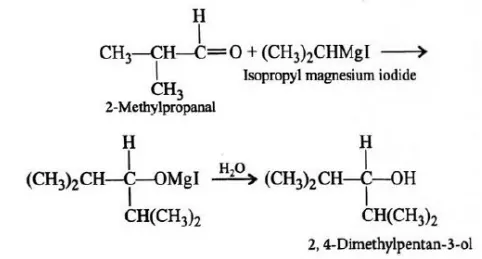CBSE Class 12 Chemistry Solved Sample Paper 2021-22 (Term-1)

41. o-hydroxy benzyl alcohol when reacted with PCl3 gives the product as (IUPAC name)
(a) o- hydroxy benzyl chloride
(b) 2- chloromethylphenol
(c) o-chloromethylchlorobenzene
(d) 4-hydroxymethylphenol
Ans 41. (b) 2- chloromethylphenol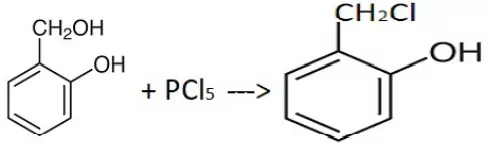42. Which of the following statements is true:
(a) Ammonia is the weakest reducing agent and the strongest base among Group 15 hydrides.
(b) Ammonia is the strongest reducing agent as well as the hydrides.
(c) Ammonia is the weakest reducing agent as well as the weakest base among Group 15 hydrides.
(d) Ammonia is the strongest reducing agent and the weakest base among Group 15 hydrides.
Ans 42. (a) Ammonia is the weakest reducing agent and the strongest base among Group 15 hydrides. The reducing character of hydrides increases down the group due to a decrease in bond dissociation enthalpy.

43. Identify the secondary alcohols from the following set:
(i) CH3CH2CH(OH)CH3
(ii) (C2H5)3COH
(iii)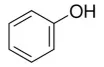(iv)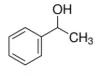(a) (i) and (iv)
(b) (i) and (iii)
(c) (i) and (ii)
(d) (i), (iii) and (iv)
Ans 43. (a)(i) and (iv)

44. Alkenes decolourise bromine water in presence of CCl4 due to the formation of
(a) Allyl bromide
(b) Vinyl bromide
(c) Bromoform
(d) Vicinal dibromide
Ans 44. (d) Vicinal dibromide CH2 = CH2 + Br2 → BrCH2 – CH2Br

CBSE Class 12 Chemistry Solved Sample Paper 2021-22 (Term-1)

45. Given below are two statements labelled as Assertion (A) and Reason (R)
Assertion (A): Electron gain enthalpy of oxygen is less than that of Flourine but greater than Nitrogen.
Reason (R): Ionisation enthalpies of the elements follow the order Nitrogen > Oxygen > Fluorine
Select the most appropriate answer from the options given below:
(a) Both A and R are true and R is the correct explanation of A
(b) Both A and R are true but R is not the correct explanation of A.
(c)A is true but R is false.
(d) A is false but R is true.
45. (c)

46. Given below are two statements labelled as Assertion (A) and Reason (R)
Assertion (A): Alkyl halides are insoluble in water.
Reason (R): Alkyl halides have halogen attached to sp3 hybrid carbon.
Select the most appropriate answer from the options given below:
(a) Both A and R are true and R is the correct explanation of A
(b) Both A and R are true but R is not the correct explanation of A.
(c) A is true but R is false.
(d) A is false but R is true.
Ans 46. (b) Assertion: Alkyl halides are insoluble in water. (correct)
Reason: Alkyl halides have halogen attached to sp3 hybrid carbon. (correct)
Alkyl halides are insoluble in water because they are unable to form hydrogen bonds with water or break pre-existing hydrogen bonds.

47. Given below are two statements labelled as Assertion (A) and Reason (R)
Assertion (A): The molarity of a solution changes with temperature.
Reason (R): Molarity is a colligative property.
Select the most appropriate answer from the options given below:
(a) Both A and R are true and R is the correct explanation of A
(b) Both A and R are true but R is not the correct explanation of A.
(c) A is true but R is false.
(d) A is false but R is true.
Ans 47. (c) Assertion: The molarity of a solution changes with temperature. (correct)
Reason: Molarity is a colligative property. (incorrect)
Molarity is a means to express concentration. It is not a physical property.

48. Given below are two statements labelled as Assertion (A) and Reason (R)
Assertion (A): SO2 is reducing while TeO2 is an oxidising agent.
Reason (R): Reducing property of dioxide decreases from SO2 to TeO2.
Select the most appropriate answer from the options given below:
(a) Both A and R are true and R is the correct explanation of A
(b) Both A and R are true but R is not the correct explanation of A.
(c) A is true but R is false.
(d) A is false but R is true.
Ans 48. (a) Assertion: SO2 is reducing while TeO2 is an oxidising agent. (correct)
Reason: Reducing property of dioxide decreases from SO2 to TeO2 (correct and reason for
Assertion)

49. Given below are two statements labelled as Assertion (A) and Reason (R)
Assertion (A): Cryoscopic constant depends on the nature of the solvent.
Reason (R ): Cryoscopic constant is a universal constant.
Select the most appropriate answer from the options given below:
(a) Both A and R are true and R is the correct explanation of A
(b) Both A and R are true but R is not the correct explanation of A.
(c) A is true but R is false.
(d) A is false but R is true.
Ans 49. (c) Assertion: Cryoscopic constant depends on the nature of the solvent. (correct)
Reason: Cryoscopic constant is a universal constant (incorrect)
Cryoscopic constant various with type of solvent.

CBSE Class 12 Chemistry Solved Sample Paper 2021-22 (Term-1)

SECTION C

This section consists of 6 multiple choice questions with an overall choice to attempt any 5. In case more than the desirable number of questions are attempted, ONLY the first 5 will be considered for evaluation.

Which of the following is the best-matched options?
(a) i-A, v- D, iii- C, iv-B
(b) i-D, ii-C, iii- A, iv-B
(c) i-D, v- D, iii- A, iv-B
(d) i-A, ii- C, iii- D, iv-B
Ans 50. (b) i-D, ii-C, iii- A, iv-B
Amino acids form proteins and exist as zwitterion, Thymine is a nitrogenous base in DNA,
Insulin is a protein, phosphodiester linkage is found in nucleic acids so also in DNA and Uracil is a nitrogenous base found in RNA which is a nucleic acid.

51. Which of the following analogies is correct:
(a) Nitrogen: 1s2 2s2 2p3 :: Argon: 1s2 2s2 2p6
(b) Carbon: maximum compounds:: Xenon: no compounds
(c) XeF2: Linear :: ClF3: Trigonal planar
(d) Helium: meteorological observations :: Argon: metallurgical processes
Ans 51. (d) Helium: meteorological observations :: Argon: metallurgical processes
Nitrogen: 1s2 2s2 2p3 :: Argon:1s2 2s2 2p6 is configuration of Neon not Argon
Carbon: maximum compounds :: Xenon: no compounds , Xenon forms compounds
XeF2: Linear :: ClF3: Trigonal planar , ClF3 is T shaped not trigonal planar

52. Complete the following analogy: Same molecular formula but different structures: A:: Non-superimposable mirror images: B
(a) A: Isomers B: Enantiomer
(b) A: Enantiomers B: Racemic mixture
(c) A: Stereoisomers B: Retention
(d) A: Isomers B: Stereoisomers
Ans 52. (a) A: Isomers B: Enantiomer
Isomers have the Same molecular formula but different structure
Enantiomers are Non-superimposable mirror images

CASE 1: Read the passage given below and answer the following questions 53-55

Early crystallographers had trouble solving the structures of inorganic solids using X-ray diffraction because some of the mathematical tools for analyzing the data had not yet been developed. Once a trial structure was proposed, it was relatively easy to calculate the diffraction pattern, but it was difficult to go the other way (from the diffraction pattern to the structure) if nothing was known a priori about the arrangement of atoms in the unit cell. It was important to develop some guidelines for guessing the coordination numbers and bonding geometries of atoms in crystals. The first such rules were proposed by Linus Pauling, who considered how one might pack together oppositely charged spheres of different radii. Pauling proposed from geometric considerations that the quality of the “fit” depended on the radius ratio of the anion and the cation.

If the anion is considered as the packing atom in the crystal, then the smaller cation fills interstitial sites (“holes”). Cations will find arrangements in which they can contact the largest number of anions. If the cation can touch all of its nearest neighbour anions then the fit is good. If the cation is too small for a given site, that coordination number will be unstable and it will prefer a lower coordination structure. The table below gives the ranges of cation/anion radius ratios that give the best fit for a given coordination geometry.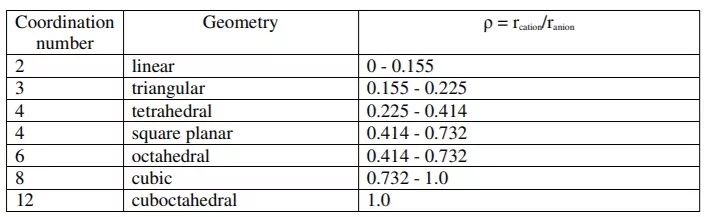53. The radius of Ag+ ion is 126pm and of I ion is 216pm. The coordination number of Ag+ ion is:

(a) 2
(b) 3
(c) 6
(d) 8

Ans 53. (c) 6 The radius of Ag+ ion is 126pm and of I ion is 216pm. The coordination number of Ag+ ion is:
ρ = rcation/ranion = 126/ 216 = 0.58 Radius ratio lies in the range 0.414 – 0.732, so has coordination number 6 or 4 according to the table.
Since none of the options is 4, so the answer is 6.

54. A solid AB has square planar structure. If the radius of cation A+ is 120pm, calculate the maximum possible value of anion B
(a) 240 pm
(b) 270 pm
(c) 280 pm
(d) 290 pm
Ans 54. (d) 290 pm
Square planar means ratio ratio is between 0.414 – 0.732
If radius of cation is 120 pm then anion should be in the range ρ = rcation/ranion
0.414 = 120/ x so x = 289.8 = 290 pm
0.732 = 120/ x so x = 163.9 = 164 pm

55. A “good fit” is considered to be one where the cation can touch:
(a) All of its nearest neighbour anions.
(b) Most of its nearest neighbour anions.
(c) Some of its nearest neighbour anions.
(d) None of its nearest neighbour anions.
Ans 55. (a) All of its nearest neighbour anions

CBSE Class 12 Chemistry Solved Sample Paper 2021-22 (Term-1)

Sample Question Paper 2021 (PDF)

SOLID STATE QUIZ QUESTIONS

https://www.sciencemotive.com/chemistryquiz/solid-state-quiz-questions/